# Common Core: 3rd Grade Math : Measurement & Data

## Example Questions

1 2 51 52 53 54 55 56 57 59 Next →

### Example Question #91 : How To Find The Perimeter Of A Figure

What is the perimeter of the regular hexagon below?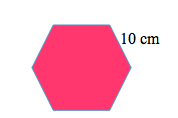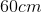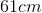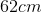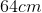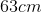Explanation:

A regular hexagon has six equal sides. To find the perimeter of a hexagon, we can multiply the known side length by(since there are six sides) or we can add the known side length to itself six times.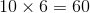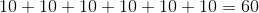### Example Question #91 : How To Find The Perimeter Of A Figure

What is the perimeter of the regular hexagon below?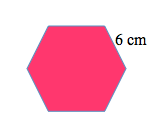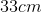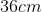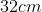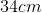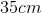Explanation:

A regular hexagon has six equal sides. To find the perimeter of a hexagon, we can multiply the known side length by(since there are six sides) or we can add the known side length to itself six times.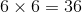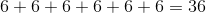### Example Question #139 : Find Perimeter Or Missing Side Lengths Of Polygons: Ccss.Math.Content.3.Md.D.8

What is the perimeter of the regular hexagon below?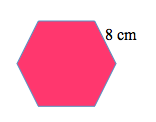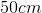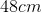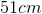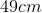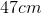Explanation:

A regular hexagon has six equal sides. To find the perimeter of a hexagon, we can multiply the known side length by(since there are six sides) or we can add the known side length to itself six times.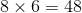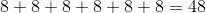### Example Question #131 : Find Perimeter Or Missing Side Lengths Of Polygons: Ccss.Math.Content.3.Md.D.8

What is the perimeter of the regular hexagon below?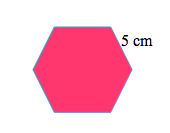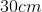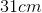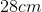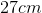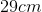Explanation:

A regular hexagon has six equal sides. To find the perimeter of a hexagon, we can multiply the known side length by(since there are six sides) or we can add the known side length to itself six times.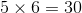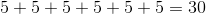### Example Question #91 : How To Find The Perimeter Of A Figure

What is the perimeter of the regular hexagon below?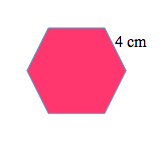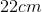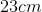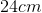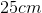Explanation:

A regular hexagon has six equal sides. To find the perimeter of a hexagon, we can multiply the known side length by(since there are six sides) or we can add the known side length to itself six times.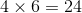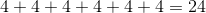### Example Question #2001 : Common Core Math: Grade 3

What is the perimeter of the regular hexagon below?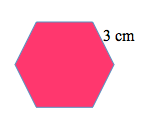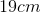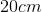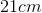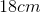Explanation:

A regular hexagon has six equal sides. To find the perimeter of a hexagon, we can multiply the known side length by(since there are six sides) or we can add the known side length to itself six times.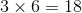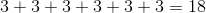### Example Question #91 : How To Find The Perimeter Of A Figure

What is the perimeter of the regular hexagon below?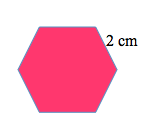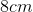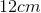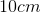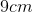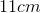Explanation:

A regular hexagon has six equal sides. To find the perimeter of a hexagon, we can multiply the known side length by(since there are six sides) or we can add the known side length to itself six times.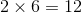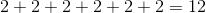### Example Question #141 : Geometric Measurement: Recognizing Perimeter

What is the perimeter of the regular hexagon below?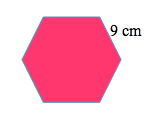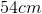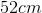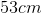Explanation:

A regular hexagon has six equal sides. To find the perimeter of a hexagon, we can multiply the known side length by(since there are six sides) or we can add the known side length to itself six times.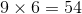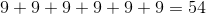1 2 51 52 53 54 55 56 57 59 Next →

### All Common Core: 3rd Grade Math Resources